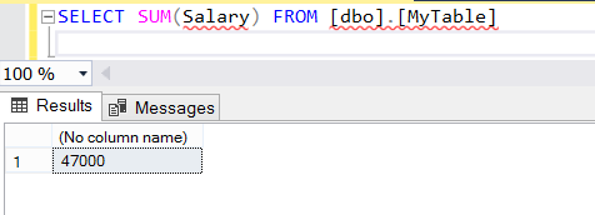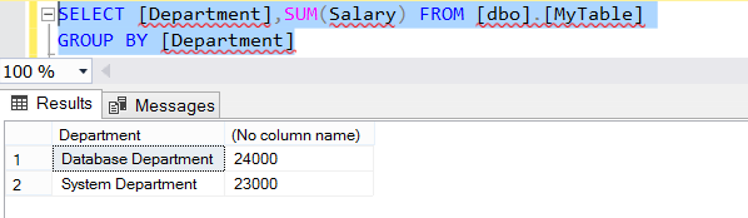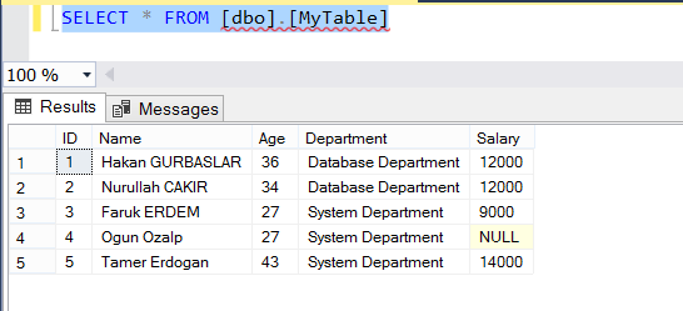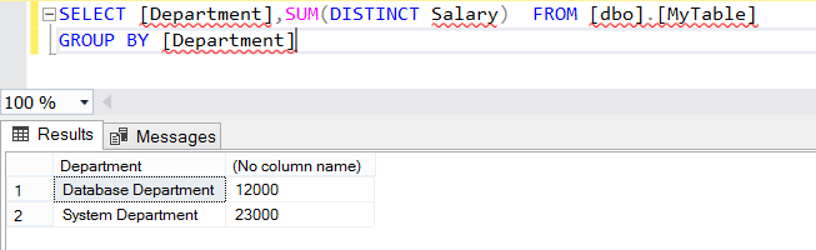Wednesday , October 5 2022

# SUM Function in SQL Server(TSQL)

We use SUM Function to calculate the sum of values in a column. SUM is a aggregated function and generally we use it with GROUP BY Clause. But sometimes we need to calculate to sum of all values in a table.

You can find the article about GROUP BY Clause below.

Let’s make examples for a better understanding of SUM Function.

Example:

First, we create a table with the help of the following script and add a few records into this table.

Calculate sum of all staff’s salary:Group the staffs accoding to their department and calculate sum of each group’s salary:We can use DISTINCT or ALL Clause with SUM Function. The default value is ALL.

If you want to calculate sum of distinct values in a dataset you can use DISTINCT Clause as follows. As you know there is two staff with the same salary in the table. First look at the all records in the table.Then Execute the previous query with the DISTINCT Clause as follows:

As a result, sum of Database Department’s Salary will return 12000, because there is two record with the same value in this group.Finally, you can use SUM Function with OVER Clause. You can find necessary details in the article “OVER Clause in SQL Server(TSQL)##### Author: dbtut

We are a team with over 10 years of database management and BI experience. Our Expertises: Oracle, SQL Server, PostgreSQL, MySQL, MongoDB, Elasticsearch, Kibana, Grafana.